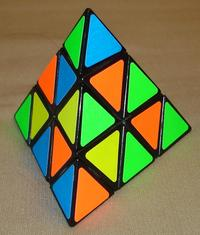# An Easy Solution for the Pyraminx Puzzle

My daughter Emily knows how to solve a Rubik-style pyramid puzzle. I tried learning her method, but I am no good at spotting the patterns that she recognizes effortlessly. Here is a simple recipe suitable for dads.With a solved puzzle or an online simulator, practice the following three moves.

1. (Rotate front face) Hold the yellow surface in front of you with the base on the bottom. Let L be the clockwise rotation about the left vertex and R be the clockwise rotation about the right vertex. L' and R' are their inverses (i.e. counterclockwise rotations). The move L R' L' R (rotate the left and right side towards you, then away from you) rotates three edges counterclockwise, flipping the colors at two of them (i.e. moving yellow to the side), but not flipping the top edge:
```
. <------ .
\       ^
flip \     /
\   / flip
V /
.
```
Do the move twice more to get back to the original.
2. (Exchange edges) With a solved pyramid, rotate the front face with L R' L' R, now physically rotate the pyramid so that the face turns by 120 degrees, then do the rotation move L R' L' R twice. Note that two edges are exchanged. To flip them back in order, orient the pyramid so that the edges to be exchanged are towards the top, do L R' L' R, physically rotate the front face 120 degrees clockwise, and do the move twice again. You now know how to exchange two edges.
3. (Bring edge down/up) Again with a solved pyramid, do L R L' R' and note how it permutes three edges: one in the middle layer, and two in the bottom layer.Do it once more and carefully look at the affected edge in the right and back. Look at its color on the bottom. Do L R L' R' a third time and notice how the bottom color becomes the left color of the top left edge. This is the move you will use to bring an edge from the bottom to the middle layer.

Those are the moves that you need to know to solve the pyramid. Here are the steps:

## Step 1. All but the Edges

Gather the three yellow middle pieces (that are neither edges nor vertices) to the front. You can do this ad-hoc, without a recipe. If necessary, twist the vertices so that the yellow edges come to the front. If necessary, rotate the back and the back vertex. Now you only need to solve six edges.

## Step 2. The Middle Edges

For each of the three middle edges locations, identify the edge that should go there. If it is not on the bottom, use the “bring edge down” move to bring it there. Then rotate the bottom face as needed. As you do that, orient the face wisely so that you get the correct color to the the bottom. If you need to rotate the edge by 120 degrees, you can leave if unflipped (moving it from the top right to the top left) or flip it (anything else). If you need to rotate the edge by 240 degrees, you can leave it unflipped (moving it from the top left and bottom to the bottom right) or flip it (anything else). Once the edge in the right place for the “bring edge up” move, put it into the middle layer.

You should now have all middle edges correctly positioned.

## Step 3. The Bottom Edges

Move the bottom face to the front. If three edges are out of place, you need to rotate them in place. If they need to be rotated clockwise, do the first move once. Now they need to be rotated counterclockwise. Figure out which edges need to be flipped, orient the front face wisely, and do the first move.

If two edges are out of place, swap them as shown above.